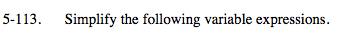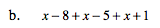### Home > MC2 > Chapter 5 > Lesson 5.3.3 > Problem5-113

5-113.
1. Simplify the following variable expressions. Homework Help ✎

1. 2x + 5 + x – 6 + 3x

2. x – 8 + x –5 + x –1Combine like terms.

2x, x, and 3x are like terms because they share the variable x to the same power.
5 and −6 are like terms because they are both constants.

2x + x + 3x = 6x and 5 + −6 = −1

6x − 1See part (a).